Next: C.2 Stack of Homogeneous Up: C.1 One Homogeneous Planar Previous: Matrix Factorization

### Propagation Matrix

Now it is easy to find a relation like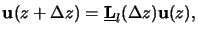(C.17)

which connects the lateral field components at two different vertical points that are at a distancez from each other. The matrix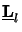(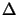z) is called the propagation matrix of the layer l and is obtained in the following way: Evaluating (C.18) at the two different points gives ul(z +z) =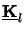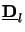(z +z)el and el =(- z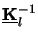ul(z), which combines with the second relation of (C.14) to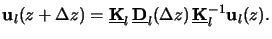(C.18)

Hence an explicit expression for the propagation matrix(z) can be derived to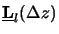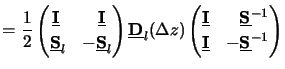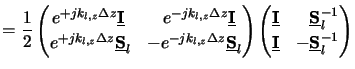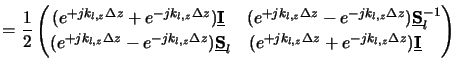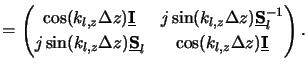(C.19)

The propagation matrix has some interesting properties. For example, in case of a vanishing distancez = 0 we obtain(0) =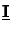. It can also be shown that the reciprocity relation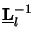(z) =(-z) exists. Additionally, in two-dimensionsa the matricesand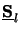are replaced by its determinants, i.e.,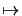det= 1 anddet= 1/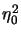. The resulting expression for the propagation matrix (C.21) can be found in many textbooks, e.g., in [11, p. 58]. Finally,(z) is a normal matrix as(z)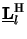(z) =(z)(z) holds, whereby the superscript H denotes Hermitian or complex-conjugate transposition. This property proves the existence of an eigenvalue decomposition. The four eigenvalues of(z) consist of two pairs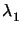and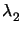that are both of manifold two. They are inverse as the determinant and thus their product equals unity, i.e., det(z) =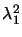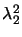= 1. The two valuesandcan easily be calculated and equal to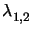= exp(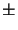jkl, zz), which correctly describes the damping exp(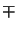Im[kl, z]z) and the oscillation exp(jRe[kl, z]z) of the plane wave traveling upwards and downwards the layer.

#### Footnotes

... two-dimensionsa
A two-dimensional analysis always suffices because the field lies in one common plane and the coordinates can be chosen in such a way that one lateral field vector component vanishes. However, we present a three-dimensional analysis as the results can directly be used as boundary conditions for the differential method.Next: C.2 Stack of Homogeneous Up: C.1 One Homogeneous Planar Previous: Matrix Factorization
Heinrich Kirchauer, Institute for Microelectronics, TU Vienna
1998-04-17